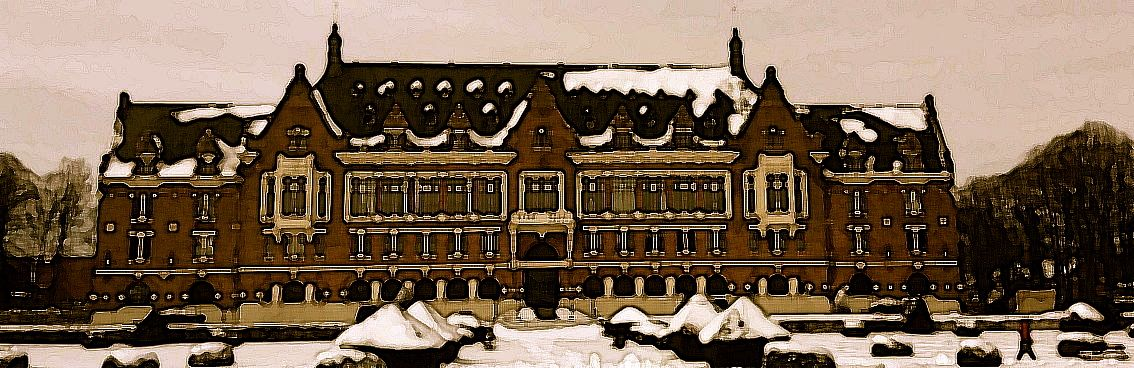## Algebra

Three main themes are studied in Algebra : firstly, quadratic form theory (splitting of quadratic and bilinear forms, isotropy of quadrics, descent for quadratic forms, Witt groups, differential forms, decomposability of central simple algebras), secondly cohomological theories on schemes (Witt groups of schems, K-théory, algebraic cobordism, homotopy theory of schemes) and finally, noncommutative algebra (ring extensions, noncommutative symmetric functions, Hopf algebras, quantum groups).

## Functional Analysis

In Functional Analysis, the two main research themes are the study of composition operators on certain types of spaces of analytic functions and the study of dynamics of linear operators (hypercyclicity, chaos, frequent hypercyclicity, ergodicity). These two themes are strongly related to the geometry of Banach spaces (or more generally Fréchet spaces), to complex analysis and to harmonic analysis.

## Geometry

Geometry covers several subjects : Algebraic geometry (rational surfaces associated to scalar and matrix elliptical solitons of KdV and Schrödinger potentials of finite-gap type and periodical doublings. Differential invariants, rank 2 vector bundles on surfaces); Mathematical physics, in particular quantum deformation, representations of Lie groups, and their applications to characterizing equivariant differential operators; Singularities (intersection (co)homologies, Poincaré duaity and singular Riemannian folliations).

## Didactics of mathematics and Histoiry of mathematics

The team Didactics of Mathematics organises its work along two directions. the first aims at producing material and at improving professionnal development of teachers (project EDU-HM). The second studies language phenomena in teaching and learning of mathematics (project LEMME).

History of mathematical theories and concepts G. Jouve takes part in the project of publishing criticized and commented complete works of D’Alembert; he is in charge of the third series. He is also interested in the history of partial differential equations from 1750 to 1850, involving pure mathematics and mathematical physics. T. Morel works on underground geometry, a practical mathematical subject initially developing in the XVIth century in german speaking areas. T. Préveraud and T. Morel study the diffusion of mathematics on long periods of time, focusing on journals. The question of translation between two mathematical and linguistic spaces is a common theme to several members of the group. It centers on the study of transfer processes, on adaptation and hybridation between the emission and the reception of the mathematical text, mainly from the.XVIIth to the XIXth century, in a context of increasing international diffusion of knowledge.